6.7 CoinFlip算法¶

6.7.1 CoinFlip算法介绍¶

问题描述：¶

E.D.Schell在1945年1月版的美国数学月刊上提出了假币问题，初始问题是给定一定数量的硬币，其中一枚假币的质量和其他真币的质量不一样（外形一样，但质量轻），给定一个天平，可用来确定哪一枚是假币（通过天平的倾斜与否来判断重量差异）。

理解问题：（以下是帮助理解规则）¶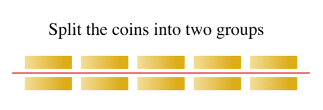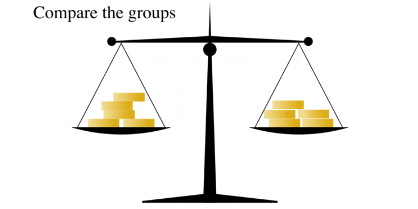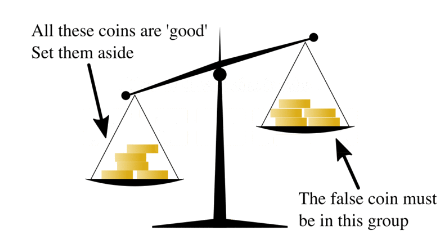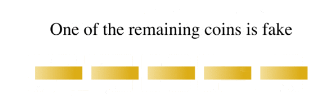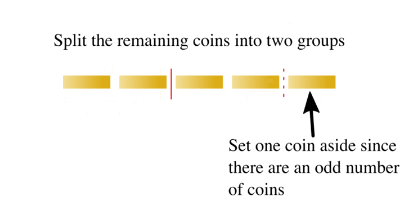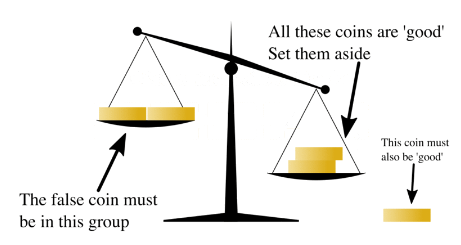1. 真币的重量均等，假币的质量也均等，假币的质量比真币轻。
2. 天平只给我们提供两个信息，平衡（两组币的重量相同）或倾斜。

量子策略模型简述：¶

\begin{split}f(x)=\left\{ \begin{aligned} & 0 & \sum_{i=1}^{n}{x_iq_i=0} \\ &1& Otherwise \\ \end{aligned} \right.\end{split}

 Results k=1 k=2 k=3 general Quantum 1 1 2<=k<=3 O(k^(1/4)) Classical logN >=2log(N/2) >=3log(N/3) Ω(k log(N/k))

参考线路图：¶